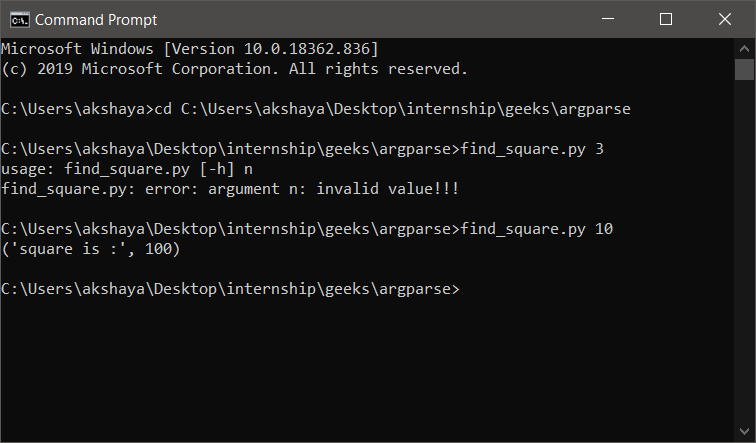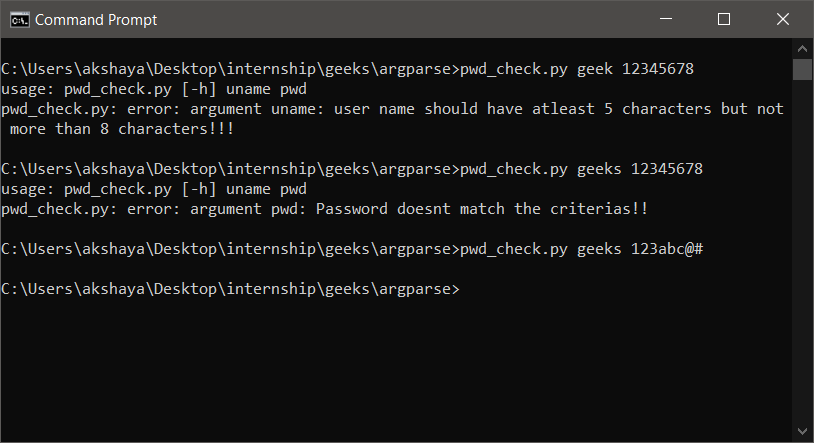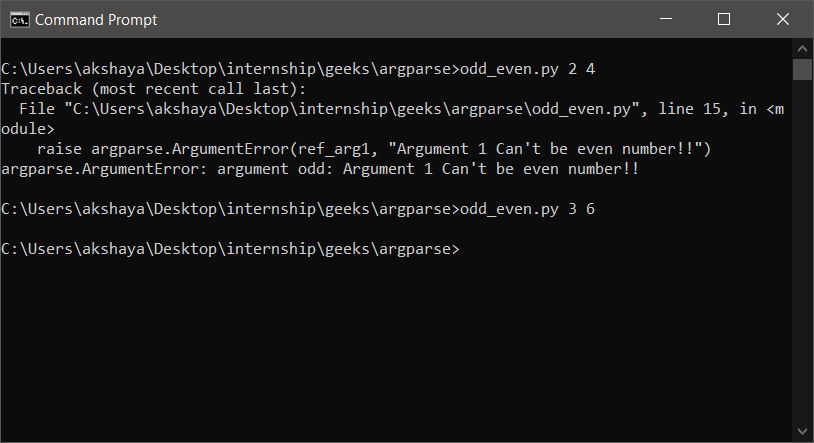Related Articles
How to handle invalid arguments with argparse in Python?
• Last Updated : 29 Aug, 2020

Argparse module provides facilities to improve the command-line interface. The methods associated with this module makes it easy to code for command-line interface programs as well as the interaction better. This module automatically generates help messages and raises an error when inappropriate arguments are passed. It even allows customizing the messages shown in case of invalid arguments.

## Ways to handle invalid arguments

1. User-defined function and ‘type’ parameter

The argparse module has a function called add_arguments() where the type to which the argument should be converted is given. Instead of using the available values, a user-defined function can be passed as a value to this parameter. This will be useful in many cases as we can define our own criteria for the argument to be valid after conversion. For example, let us take a simple example. One has to enter a number in the range 5 to 15, if it exceeds, then an error has to be raised and that too immediately after the conversion. Otherwise, the square of the number will be displayed. Look at the python code below which is saved as find_square.py

## Python

 `import` `argparse ` ` `  `# function to convert the input and  ` `# check the range ` `def` `checker(a): ` `    ``num ``=` `int``(a) ` `     `  `    ``if` `num < ``5` `or` `num > ``15``: ` `        ``raise` `argparse.ArgumentTypeError(``'invalid value!!!'``) ` `    ``return` `num ` ` `  ` `  `parser ``=` `argparse.ArgumentParser( ` `    ``description``=``'Processing integers in range 5 to 15'``) ` ` `  `# passing the function for 'type' parameter ` `parser.add_argument(``'n'``, ``type``=``checker) ` ` `  `res ``=` `parser.parse_args() ` `print``(``"square is :"``, res.n``*``res.n) `

Output:find_square.py output

If the argument passed is not in the given range, for example, the argument given for the first time the image above ‘3‘, the error message invalid value!!! is displayed. The argument passed next time is 10 which is in the specified range and thus the square of 10 is printed.

Now, look at another example, where you don’t want to type convert the input but specify some criteria so that it could be a valid input. The program given below takes two arguments – uname, pwd (user name, password). Here the criteria for setting username is, it should have at least 5 characters but not more than 8. The password must have at least 1 digit, at least 2 alphabets, and at least 1 special character. The parser is set to process in such a way and the program is stored as pwd_check.py.

## Python

 `import` `argparse ` ` `  `# function to check user name ` `def` `checker(a): ` `    ``if` `len``(a) > ``8` `or` `len``(a) < ``5``: ` `        ``raise` `argparse.ArgumentTypeError( ` `            ``'user name should have atleast ``5` `characters \ ` `            ``but ``not` `more than ``8` `characters!!!') ` `    ``return` ` `  `# function to check password ` `def` `checker_pwd(b): ` ` `  `    ``d ``=` `0`  `# initial count of digits ` `    ``a ``=` `0`  `# initial count of alphabets ` `    ``ss ``=` `0`  `# initial count of special characters ` ` `  `    ``for` `i ``in` `range``(``0``, ``len``(b)): ` `      ``# increment alphabet count ` `        ``if` `(b[i] >``=` `'a'` `and` `b[i] <``=` `'z'``) ``or` `(b[i] >``=` `'A'` `and` `b[i] <``=` `'Z'``): ` `            ``a ``=` `a``+``1` ` `  `      ``# increment digit count ` `        ``elif` `(b[i] >``=` `'0'` `and` `b[i] <``=` `'9'``): ` `            ``d ``=` `d``+``1` ` `  `       ``# increment special characters count ` `        ``else``: ` `            ``ss ``=` `ss``+``1` ` `  `    ``# check criteria ` `    ``if` `d < ``1` `or` `a < ``2` `or` `ss < ``1` `or` `len``(b) > ``6``: ` `        ``raise` `argparse.ArgumentTypeError( ` `            ``'Password doesnt match the criterias!!'``) ` `    ``return` ` `  ` `  `parser ``=` `argparse.ArgumentParser() ` `parser.add_argument(``'uname'``, ``type``=``checker) ` `parser.add_argument(``'pwd'``, ``type``=``checker_pwd) ` `parser.parse_args() `

Output:pwd_check.py output

• First run: the arguments are geek 12345678. The username should have at least 5 characters.
• Second run: the arguments are geeks 12345678. The password’s criterion is not satisfied.
• Third run: the arguments are geeks 123abc@#. These pass all the constraints and so the system exits

2. Using ArgumentError()

This function takes up two parameters namely the argument and the message to be displayed. To use this a variable that refers to the argument should be set up. Look at the example below. The program takes two values from the user, first should be an odd number, and second should be an even number. It is saved as odd_even.py.

## Python

 `import` `argparse ` ` `  `parser ``=` `argparse.ArgumentParser() ` ` `  `# a variable to hold odd numbers ` `ref_arg1 ``=` `parser.add_argument(``'odd'``, ``type``=``int``) ` ` `  `# a variable to hold even number ` `ref_arg2 ``=` `parser.add_argument(``'even'``, ``type``=``int``) ` ` `  `args ``=` `parser.parse_args() ` ` `  `# raising error in cas of ` `if` `args.odd ``%` `2` `=``=` `0``: ` `    ``raise` `argparse.ArgumentError(ref_arg1, "Argument ``1` `Can't \ ` `    ``be even number!!") ` ` `  `if` `args.even ``%` `2` `!``=` `0``: ` `    ``raise` `argparse.ArgumentError(ref_arg1, "Argument ``2` `Can't be\ ` `    ``odd number!!") `

Output:odd_even.py output

Attention geek! Strengthen your foundations with the Python Programming Foundation Course and learn the basics.

To begin with, your interview preparations Enhance your Data Structures concepts with the Python DS Course.

My Personal Notes arrow_drop_up
Recommended Articles
Page :